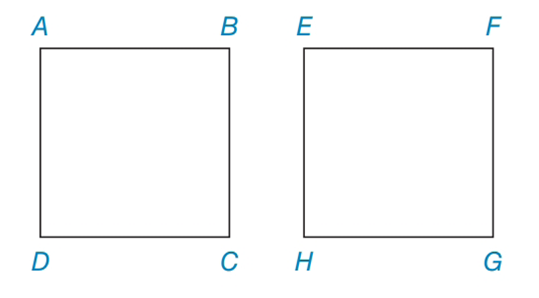Chapter 4.3, Problem 31EElementary Geometry For College St...

7th Edition
Alexander + 2 others
ISBN: 9781337614085

Solutions

Chapter
SectionElementary Geometry For College St...

7th Edition
Alexander + 2 others
ISBN: 9781337614085
Textbook Problem

Are quadrilaterals ABCD and EFGH congruenta) if both are squares and A B ¯ ≅ E F ¯ ? b) if each is a rhombus and A B ¯ ≅ E F ¯ ?To determine

(a)

To find:

AB¯EF¯, if both are squares.

Explanation

The quadrilateral if all the sides are equal it is called square or rhombus.

The properties of square:

1) All the properties of a rhombus apply (the ones that matter here are parallel sides, diagonals are perpendicular bisects of each other, and diagonals bisect the angles).

2) All the properties of a rectangle apply (the only one that matters here is diagonals are congruent).

3) All sides are congruent.

4) All angles are right angles.

Calculation:

Given:

Consider quadrilaterals ABCD and EFGH.

Let us take both ABCD and EFGH are squares.

Step 1:

Now we have to find both squares are congruent or not.

All squares are not congruent. But all squares are similar.

Two polygons are similar if the angles of one polygon have an exact match in the other polygon, and the ratio of corresponding side lengths between the two polygons is constant.

This is true of all squares, since all squares have four 90-degree angles, and the ratio of the length of a side of the two squares is identical.

So, both the squares ABCD and EFGH are congruent...

To determine

(b)

To find:

AB¯EF¯, if both are rhombus.

Still sussing out bartleby?

Check out a sample textbook solution.

See a sample solution

The Solution to Your Study Problems

Bartleby provides explanations to thousands of textbook problems written by our experts, many with advanced degrees!

Get Started

Calculate y'. 21. y = 3x ln x

Single Variable Calculus: Early Transcendentals, Volume I

In Exercises 3-11, find the derivative of the function. f(x)=12x

Calculus: An Applied Approach (MindTap Course List)

The fourth term of {an} defined by a1 = 3, and for n = 1, 2, 3, … is:

Study Guide for Stewart's Single Variable Calculus: Early Transcendentals, 8th